Latest Banking jobs   »

# Quantitative Aptitude Quiz For IBPS RRB PO/Clerk Mains 2022- 26th August

Q1. Abhi invested some amount on scheme ‘P’ which offer CI at the rate of 15% p.a.. After 2 years he got Rs. 1032 as interest. Abhi invest the amount he got from scheme ‘P’ in scheme ‘Q’ which offer 15% p.a. at SI for 4 years. Find the total interest he earned from scheme P and scheme Q together?
(a) 3571.2
(b) 3715.2
(c) 4232
(d) 3148
(e) 3379.2

Q2. S₁ is a series of five consecutive multiple of three, whose sum is 180 and S₂ is the series of four consecutive multiple of four whose second smallest number is 13 more than second highest number of S₁ series. Find the average of smallest number of S₁ series and highest number of S₂ series.
(a) 51
(b) 49
(c) 47
(d) 45
(e) 43

Q3. Aman is 50% more efficient than Ravi while 20% more efficient than Mohan. If Ravi and Aman together can complete a work in 18 days, then in what time Ravi and Mohan can complete the same work if they work alternatively starting from Ravi ?
(a)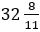days
(b) 20 days
(c) 40 days
(d) 36 days
(e) 30 days

Q4. A milkman mixed 32 litre of water in 48 litre of milk and sold 30 litre of mixture. Then he again added some milk and water whose quantity is in the ratio 5 : 4 in the remaining mixture so that ratio of water and milk become 7 : 10. Now he sell 25% of mixture, then find the amount of milk in the rest of the mixture.
(a) 30 liter
(b) 21 liter
(c) 15 liter
(d) 22.5 liter
(e) 24 liter

Q5. A man swimming at 75 percent of his usual speed can covers 128 km in 8 hours in downstream. In the return journey he swims at 50% of his usual speed and take 32 hours. Speed of man is what percent more than speed of stream?
(a) 100%
(b) 200%
(c) 300%
(d) 400%
(e) 500%

Q6. Aman invest Rs. (x – 2000) in scheme ‘P’ which offers 20% CI pa while Rs. (x + 2000) in scheme ‘Q’ which offers 8% SI pa. After 2 year difference between interest earn from scheme P and Q is 1600, then amount invested by Aman in scheme ‘Q’ is what percent more/less than amount invested by him in scheme ‘P’ ?
(a) 75%
(b) 133⅓%
(c) 150%
(d) 50%
(e) 33⅓%

Q7. Two Beaker A and B having mixture of milk and water in ratio of 3 : 2 and 2 : 3 respectively. 20 liter of mixture is taken out from A and mix in beaker B, due to which new ratio of milk and water in B becomes 11 : 14 and volume of beaker B become equal to initial volume of A. Now, ‘x’ liter mixture is taken out from B and mix in A and ratio of milk and water become 7 : 6 in beaker A. Find the value of ‘x’.
(a) 20
(b) 25
(c) 50
(d) 30
(e) 40

Q8. Volume of a cone and cylinder, having same base radius, are in ratio of 4 : 3. If ratio of height to that of and radius of cone is 4 : 3 then find the ratio of total surface area of cone and that of cylinder.
(a) 1 : 1
(b) 3 : 1
(c) 5 : 3
(d) 2 : 1
(e) 4 : 1

Q9. Ratio of markup price and selling price when a discount is given, of an article is 5 : 4 and ratio of cost price and selling price is 5 :6. If discount% is double up then a loss of 60 Rs. occur. Find the mark up price.
(a) 600
(b) 750
(c) 840
(d) 900
(e) 720

Q10. A bus and a car start from a place A toward B simultaneously at 10:00 am. Bus reach at B in 4 hours and return for C, which is exactly in the middle of A and B, and meet the car after 1 hour after leaving B. Find the time at which car reach C after returning from B.
(a) 6:00 pm
(b) 8:00 pm
(c) 10:00 pm
(d) 8:00 am
(e) 12:00 pm

Q11. Ratio of numerical value of rate of interest and time period is 4 : 1. Man invested Rs. 2400 and gets Rs. 864 as simple interest. Find the value of X, if man invested Rs. (2400 + X) at same rate of interest on C.I. for two years and get Rs. 814.08 as interest?
(a) 600 Rs.
(b) 800 Rs.
(c) 400 Rs.
(d) 200 Rs.
(e) 540 Rs.

Q12. Ratio of speed of faster train to slower train is 9 : 7 and length of two trains is 120 m and 180 m respectively. If both trains increased their respective speed by 25% and then cross each other in 36 sec running in same direction, then find in what time both trains cross each other running in opposite direction with their usual speed?
(a) 64/6 sec
(b) 58/6 sec
(c) 52/6 sec
(d) 49/8 sec
(e) 45/8 sec

Q13. A and B entered into a business with the capital of Rs. (X + 1200) and Rs (X+1500) respectively. After eight months from starting A withdraw half of his investment and B doubled his investment. If at the end of the year A got Rs. 4250 as profit share out of total profit of Rs. 11250, then find three times of initial investment of B ?
(a) Rs 32,400
(b) Rs 30,750
(c) Rs 30,500
(d) Rs 31,500
(e) Rs 31,770

Q14. Two farmers A and B have mixture of potassium and Urea in the quantity of (θ + 54) kg & (θ + 84) kg respectively. The ratio of potassium and Urea in mixture which farmer A has is 3 : 2, while farmer B has 2 : 1. If 60% and 66 ⅔% of their respective mixture farmer A and B used for their field and the remaining mixture of A and B are equal, then find the quantity of potassium in the mixture of farmer B has ?
(a) 60 kg
(b) 120 kg
(c) 90 kg
(d) 100 kg
(e) 75 kg

Q15. A bag contains seven red balls, ‘a’ green balls & ‘b’ yellow balls. If one ball taken out from bag, then probability of being it green is 5/16, while being it yellow is ¼. Find difference between number of green and yellow balls in that bag?
(a) 1
(b) 0
(c) 2
(d) 4
(e) 3

Solutions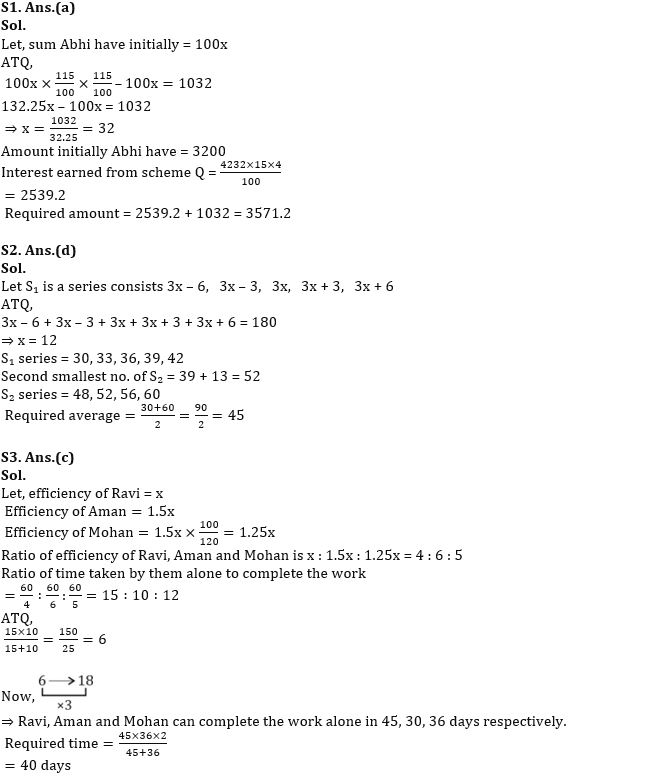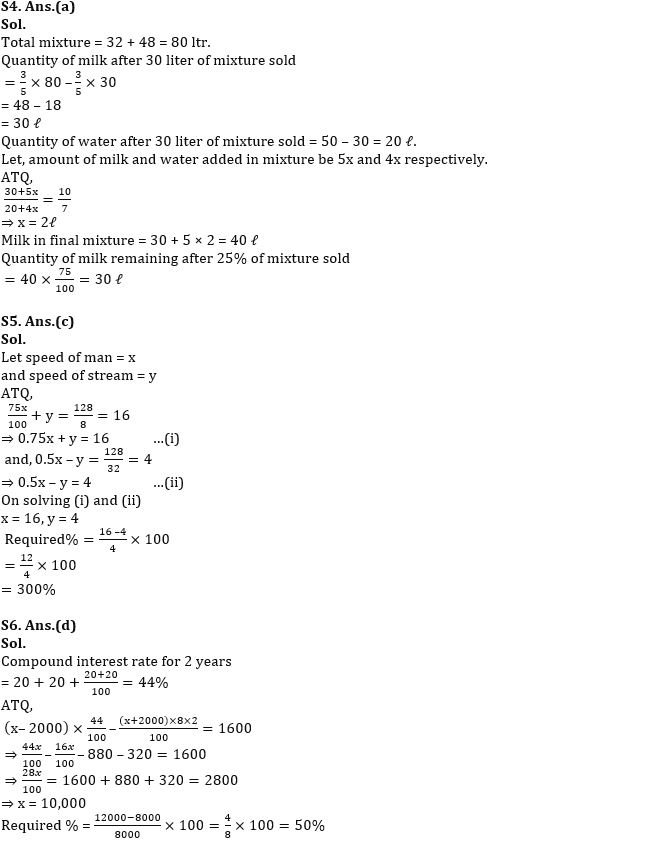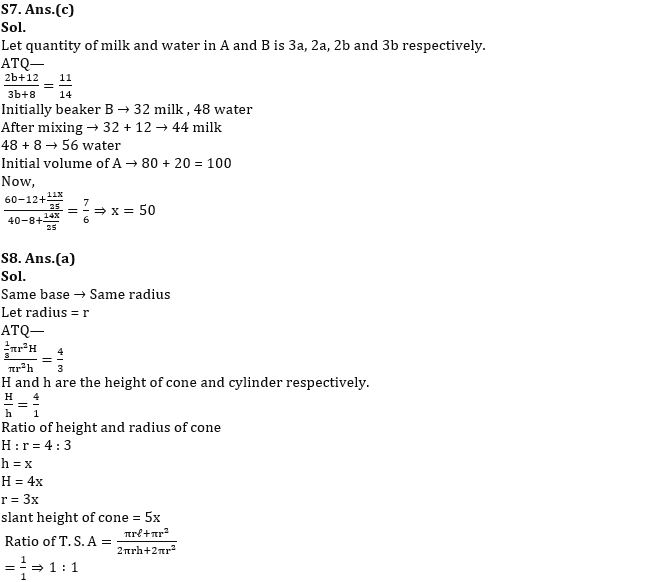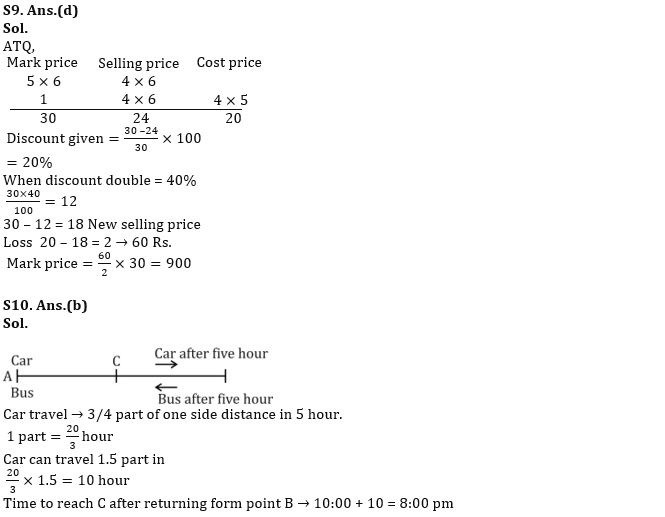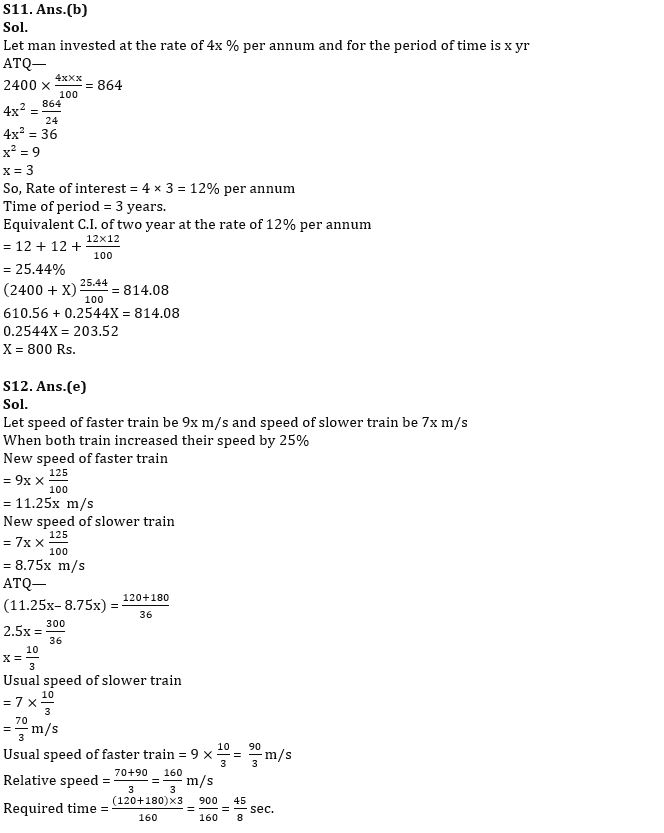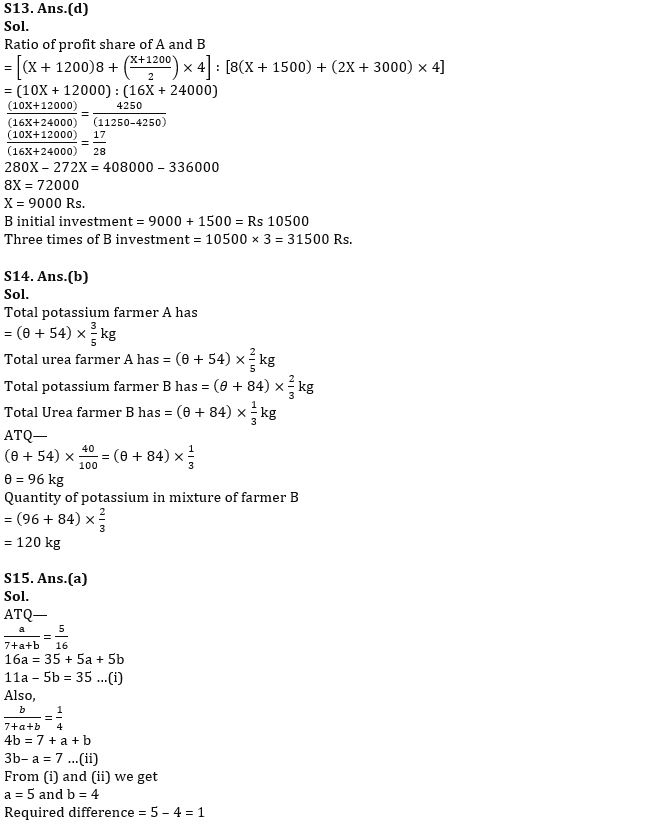#### Congratulations!Download Hindu Review of October 2021: Free PDF